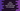# Java program to calculate the area and perimeter of a rectangle### Java Program to calculate the area and perimeter of a rectangle :

In this tutorial, we will learn how to find the area and perimeter of a rectangle. To find the area and perimeter, we need only height and width of the rectangle. Area of a rectangle is_ ‘height * width’_ and perimeter is ’ 2* ( height + width )’ .

Let’s take a look into the program :

### Java program :

``````import java.util.Scanner;

public class Main {

//8

/**
* Utility function for System.out.println
*
* @param message : string to print
*/
private static void println(String message) {
System.out.println(message);
}

/**
* Utility function for System.out.print
*
* @param message : string to print
*/
private static void print(String message) {
System.out.print(message);
}

/**
* main method
*
* @throws java.lang.Exception
*/
public static void main(String[] args) throws java.lang.Exception {
//1
double height, width, area, perimeter;

//2
Scanner scanner = new Scanner(System.in);

//3
print("Enter the height of the rectangle : ");
height = scanner.nextFloat();

print("Enter the width of the rectangle : ");
width = scanner.nextFloat();

//4
area = height * width;
perimeter = 2 * (height + width);

//5
println("Area = " + area);
println("Perimeter = " + perimeter);

}

}``````

### Sample Output :

``````Enter the height of the rectangle : 10
Enter the width of the rectangle : 10
Area = 100.0
Perimeter = 40.0

Enter the height of the rectangle : 9
Enter the width of the rectangle : 15
Area = 135.0
Perimeter = 48.0``````

### Explanation :

1. Create variables to store height, width, area and perimeter of the rectangle.
2. Create one ’Scanner’ object to scan the values.
3. Read the height and width of the rectangle.
4. Find the area and perimeter and save the values in variables ’area’ and ’perimeter‘.
5. Print out ’Area’ and ’Perimeter